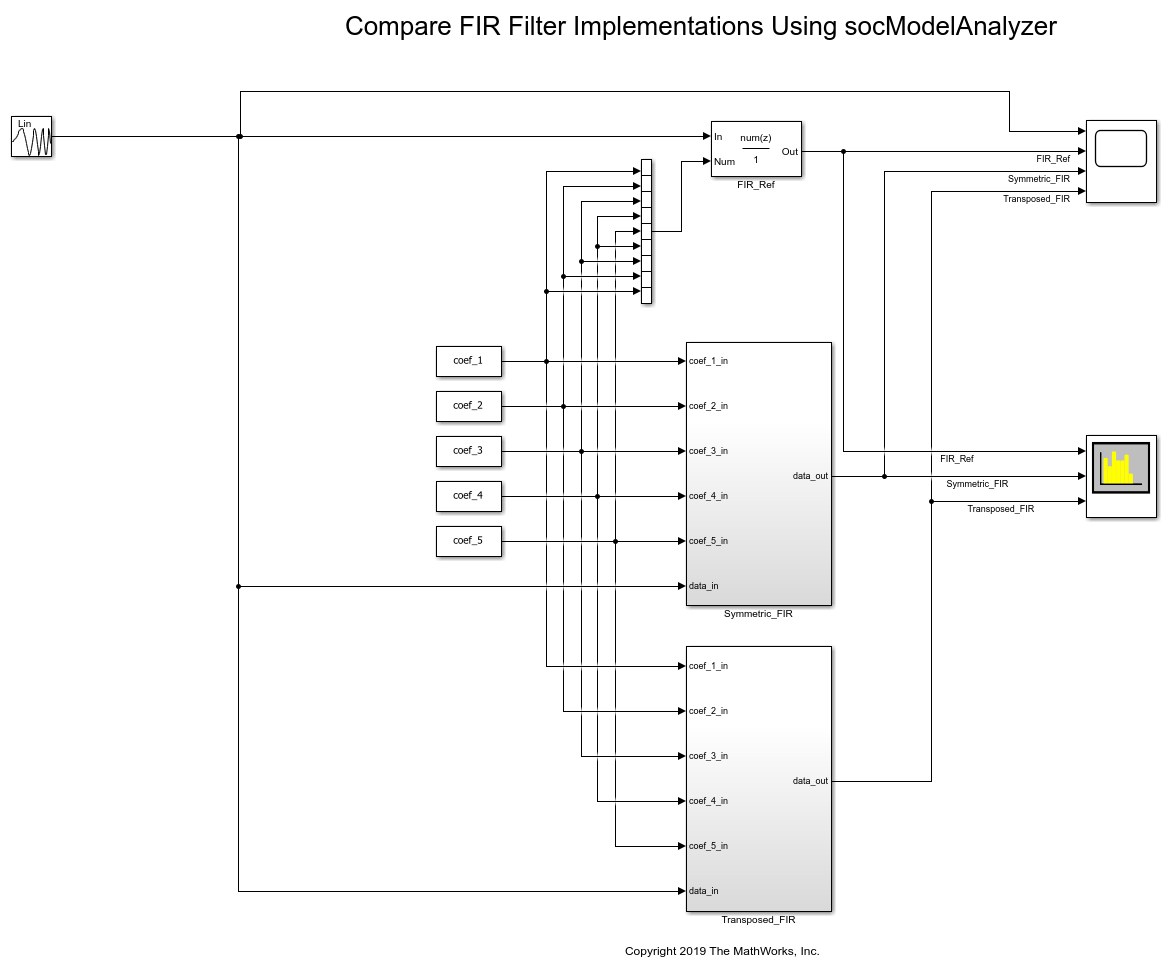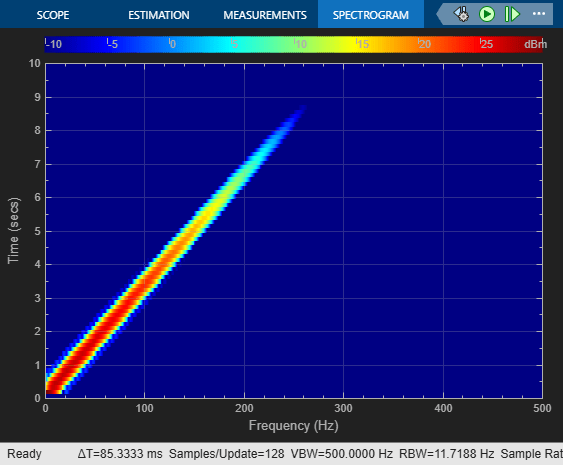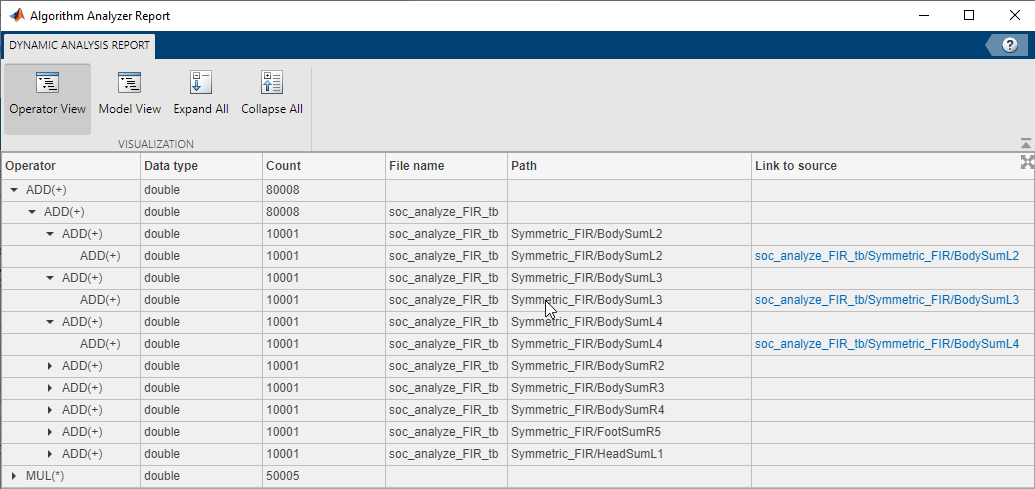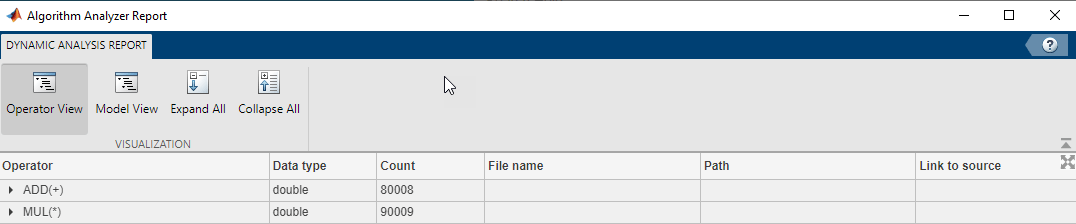# Compare FIR Filter Implementations Using socModelAnalyzer

This example shows how to analyze and compare different implementations of a Simulink algorithm based on number of arithmetic operations. Use SoC Blockset's `socModelAnalyzer` function to generate reports showing the number of add and multiply operators for different implementations of FIR Filter using static and runtime execution.

The design task is to evaluate two implementations of a FIR filter and compare the implementation costs. This example uses the number of operators as a way to measure implementation cost.

In order to meet system requirements such as speed, latency, and hardware resources, several implementations of the algorithm are usually considered and compared. It is helpful to know the number of arithmetic operators used in an implementation in order to understand resource usage and allocation.

Manual analysis and calculation of the number of arithmetic operators is tedious, error prone and time consuming. Manual calculations can be inaccurate for an algorithm involving a branch, loop or recursion construct, and might be impossible to calculate if the execution path depends on the input data or random factors (for example: a convergence algorithm).

### Structure of Model

In the soc_analyze_FIR_tb model, a low pass digital FIR Filter is implemented in two ways. Symmetric_FIR subsystem exploits symmetry in coefficients to optimize the resources while the Transposed_FIR subsystem employs a filter structure geared towards higher speed of operation. A chirp input signal is used as an input stimulus and a FIR_ref (Discrete FIR Filter) block is used as a reference for checking numerical correctness of the implementations.

Open the `soc_analyze_FIR_tb` model in Simulink and examine the structure of the model.

```open_system('soc_analyze_FIR_tb'); ```The design of low pass FIR filter is carried out using the `filterDesigner` app to generate coefficients for an 8th order FIR filter. The FIR filter has a cutoff frequency at 0.25 (normalized) and a passband ripple and stop band attenuation of 1dB and 60 dB respectively. These coefficients are set in the model via the model initialize callback.

Simulate the model to validate the functionality of both implementations against the reference FIR block. Observe that the responses of filter implementations match with the reference.

```sim('soc_analyze_FIR_tb'); ```### Compare Implementations Using Algorithm Analyzer

Use socModelAnalyzer to generate reports for the number of arithmetic operators in each implementation, and compare the implementations. The reports are generated using the runtime execution of the model.

Symmetric FIR filter:

To estimate the number of operators for Symmetric FIR filter implementation, use `socModelAnalyzer` function specifying its subsystem name for `IncludeBlockPath` property. Set the output folder to specify where reports will be generated. Execute the following command:

```socModelAnalyzer('soc_analyze_FIR_tb.slx','Folder','report_sym','IncludeBlockPath','soc_analyze_FIR_tb/Symmetric_FIR'); ```
```Generating operators analysis report for \\fs-58-ah\vmgr\$\home08\jchevali\Documents\MATLAB\Examples\soc-ex82446029\soc_analyze_FIR_tb.slx ... Saving report files in \\fs-58-ah\vmgr\$\home08\jchevali\Documents\MATLAB\Examples\soc-ex82446029\report_sym. Operator estimate: <a href="matlab: socAlgorithmAnalyzerReport('\\fs-58-ah\vmgr\$\home08\jchevali\Documents\MATLAB\Examples\soc-ex82446029\report_sym\soc_analyze_FIR_tb.mat')">Open report viewer</a> Done. ```Open the report by clicking on the Open report viewer link on the MATLAB console. Alternatively, you can use the `socAlgorithmAnalyzerReport` function. The viewer provides two views. The first view is the Operator view which presents the data such that each row corresponds to an operator. The second view is the Model view where each row corresponds to a Simulink subsystem path. You can toggle between the two views using the buttons on the toolstrip on the viewer. Additionally, reports are saved in the report_sym folder as a MAT file (soc_analyze_FIR_tb.mat) and an Excel® file (soc_analyze_FIR_tb.xlsx).

By default, the viewer opens the Operator view. It opens the aggregate view of each operator and data type. For example in the Symmetric FIR filter there are a total of 8 additions `ADD(+)` of type double and 5 multiplications `MUL(*)` of type double executed 10001 times each. (The model simulation duration is 10s and the base rate is 10ms: this produces 10000 simulation cycles plus 1 for initialization.) To get the detailed report for each operator, expand it to another level. The viewer will show the operator count as used in various blocks. Trace the operator by clicking on one of the links in the last column of the report to highlight the location of the operator in the soc_analyze_FIR_tb model.Transposed FIR filter:

To estimate the number of operators for Transposed FIR filter implementation, use the `socModelAnalyzer` function specifying `'soc_analyze_FIR_tb/Transposed_FIR'` for the 'IncludeBlockPath' property. Set the output folder to `report_trans`, where the reports will be generated. Execute the following command:

```socModelAnalyzer('soc_analyze_FIR_tb.slx','Folder','report_trans','IncludeBlockPath','soc_analyze_FIR_tb/Transposed_FIR'); ```
```Generating operators analysis report for \\fs-58-ah\vmgr\$\home08\jchevali\Documents\MATLAB\Examples\soc-ex82446029\soc_analyze_FIR_tb.slx ... Saving report files in \\fs-58-ah\vmgr\$\home08\jchevali\Documents\MATLAB\Examples\soc-ex82446029\report_trans. Operator estimate: <a href="matlab: socAlgorithmAnalyzerReport('\\fs-58-ah\vmgr\$\home08\jchevali\Documents\MATLAB\Examples\soc-ex82446029\report_trans\soc_analyze_FIR_tb.mat')">Open report viewer</a> Done. ```Open the report for Transposed FIR filter by clicking on the Open report viewer link on the MATLAB console.For the Transposed FIR filter the report shows an estimated number of 8 additions of type double and 9 multiplications of type double (each of them executed 10001 times).

Comparison of Symmetric and Transposed Implementations:

As per above reports obtained by socModelAnalyzer, Symmetric FIR filter uses fewer multiplication operators (9) than the Transposed FIR filter (5). They both use the same number of add operators (8).

### Conclusion

You used the `socModelAnalyzer` function to estimate and analyze the number of arithmetic operators in two FIR filter implementations. You generated operator reports for both Symmetric and Transposed FIR filters. You compared the number of multiply and add operators for two implementations.

You can use socModelAnalyzer for analyzing the number of operators in your own Simulink algorithm.

﻿
##### Support